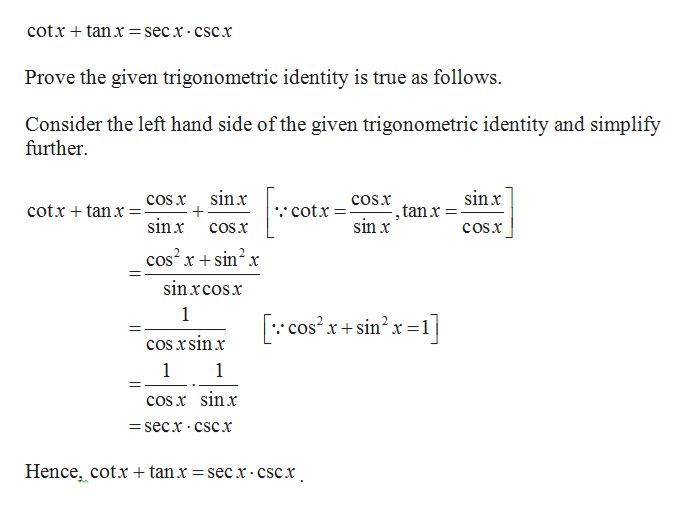# (5) Verify the following identities.(a) cot x + tan x = sec x csc x(b) sec4 x − sec2 x = tan4 x + tan2 x

Question
2 views

(5) Verify the following identities.
(a) cot x + tan x = sec x csc x
(b) sec4 x − sec2 x = tan4 x + tan2 x

check_circle

Step 1

(a) The given trigonomet...help_outlineImage Transcriptionclosecotxtanx = secx cscx Prove the given trigonometric identity is true as follows Consider the left hand side of the given trigonometric identity and simplify further sinx sinx cOs x cosx cotxtanx = cotx tanx sinx sin x cosx cosx cos2 rsin2 x sinxcosx 1 cos2xsin2x=1 cos xsinx 1 1 cos x sinx =secx CSCx Hence, cotx + tan x = sec x cscx fullscreen

### Want to see the full answer?

See Solution

#### Want to see this answer and more?

Solutions are written by subject experts who are available 24/7. Questions are typically answered within 1 hour.*

See Solution
*Response times may vary by subject and question.
Tagged in

### Other